Chapter 9 Class 11 Sequences and Series

Class 11
Important Questions for exams Class 11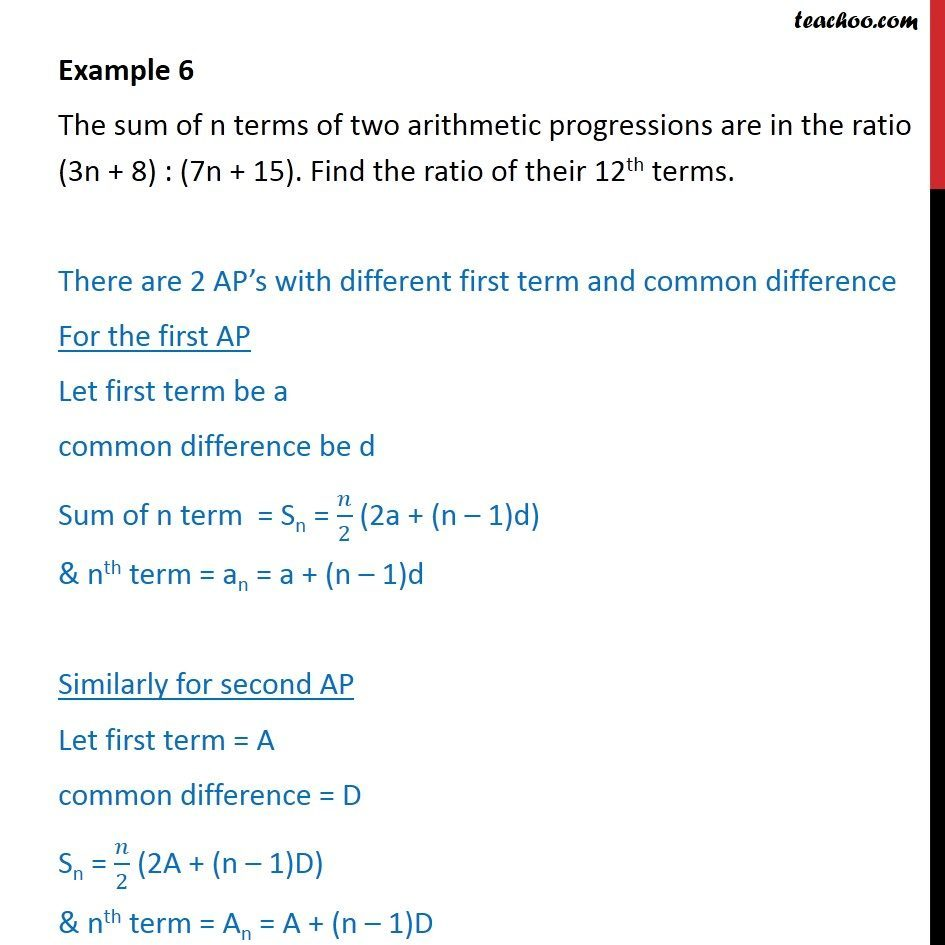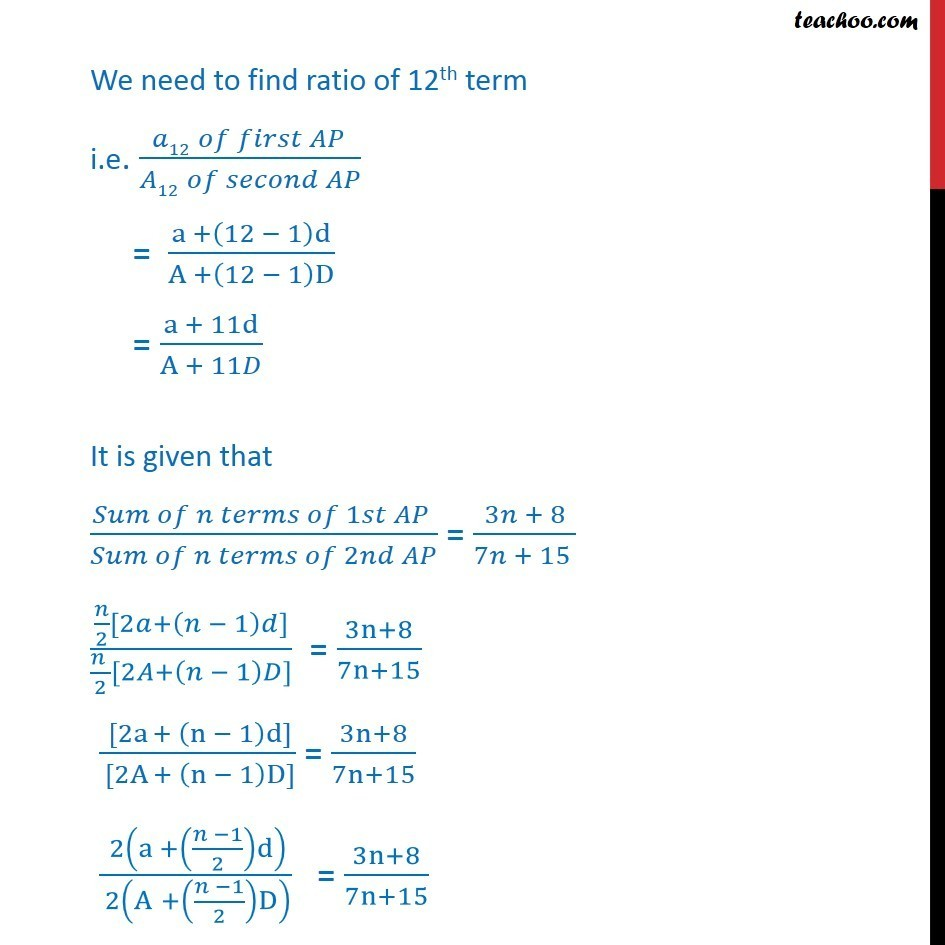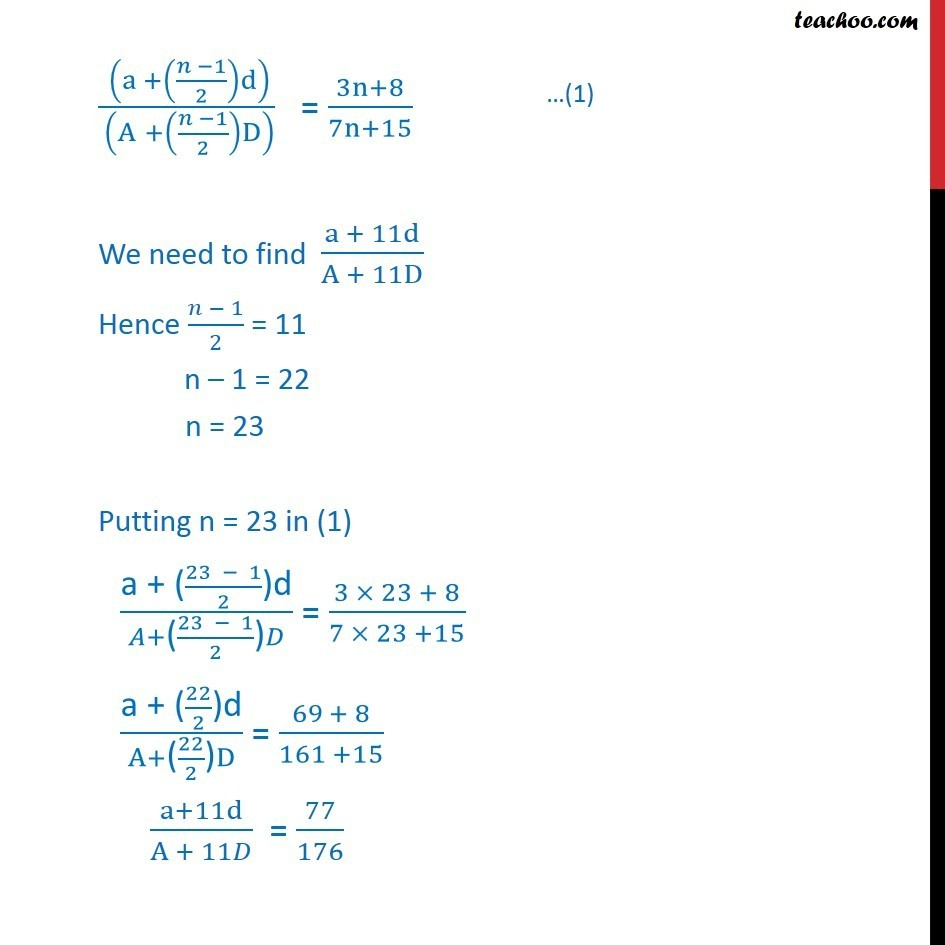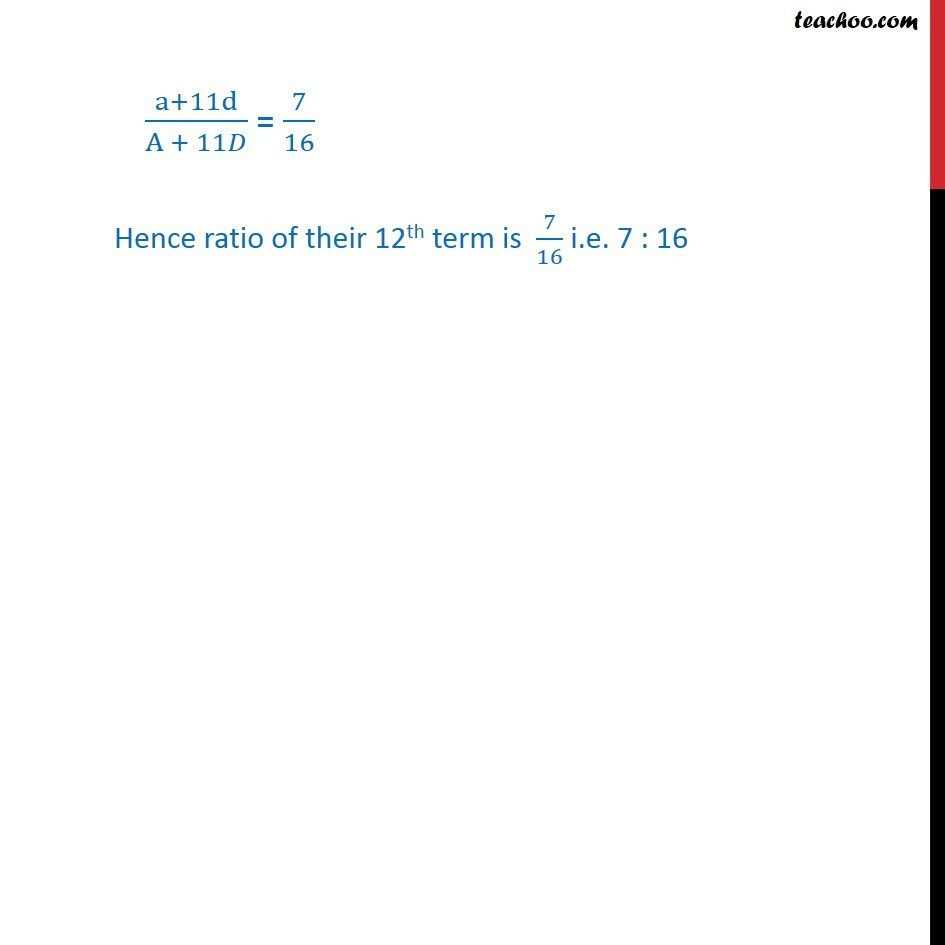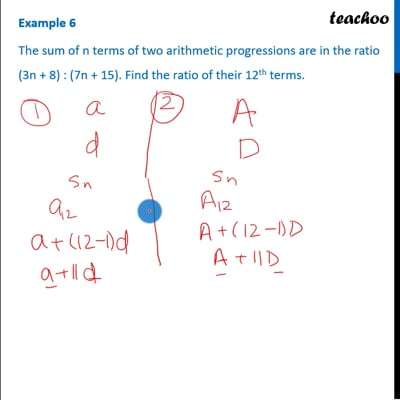This video is only available for Teachoo black users

Solve all your doubts with Teachoo Black (new monthly pack available now!)

### Transcript

Example 6 The sum of n terms of two arithmetic progressions are in the ratio (3n + 8) : (7n + 15). Find the ratio of their 12th terms. There are 2 AP s with different first term and common difference For the first AP Let first term be a common difference be d Sum of n term = Sn = /2 (2a + (n 1)d) & nth term = an = a + (n 1)d Similarly for second AP Let first term = A common difference = D Sn = /2 (2A + (n 1)D) & nth term = An = A + (n 1)D We need to find ratio of 12th term i.e. ( 12 )/( 12 ) = (a +(12 1)d)/(A +(12 1)D) = (a + 11d)/(A + 11 ) It is given that ( 1 )/( 2 ) = (3 + 8)/(7 + 15 ) ( /2[2 +( 1) ])/(( )/2[2 +( 1) ]) = (3n+8)/(7n+15) ( [2a + (n 1)d])/( [2A + (n 1)D]) = (3n+8)/(7n+15) ( 2(a +(( 1)/2)d))/( 2(A +(( 1)/2)D) ) = (3n+8)/(7n+15) ( (a +(( 1)/2)d))/( (A +(( 1)/2)D) ) = (3n+8)/(7n+15) We need to find (a + 11d)/(A + 11D) Hence ( 1)/2 = 11 n 1 = 22 n = 23 Putting n = 23 in (1) ("a + (" (23 1)/2 ")d" )/( +"(" (23 1)/2 ")" ) = (3 23 + 8)/(7 23 +15) ("a + (" 22/2 ")d" )/(A+"(" 22/2 ")" D) = (69 + 8)/(161 +15) (a+11d)/(A + 11 ) = 77/176 (a+11d)/(A + 11 ) = 7/16 Hence ratio of their 12th term is 7/16 i.e. 7 : 16